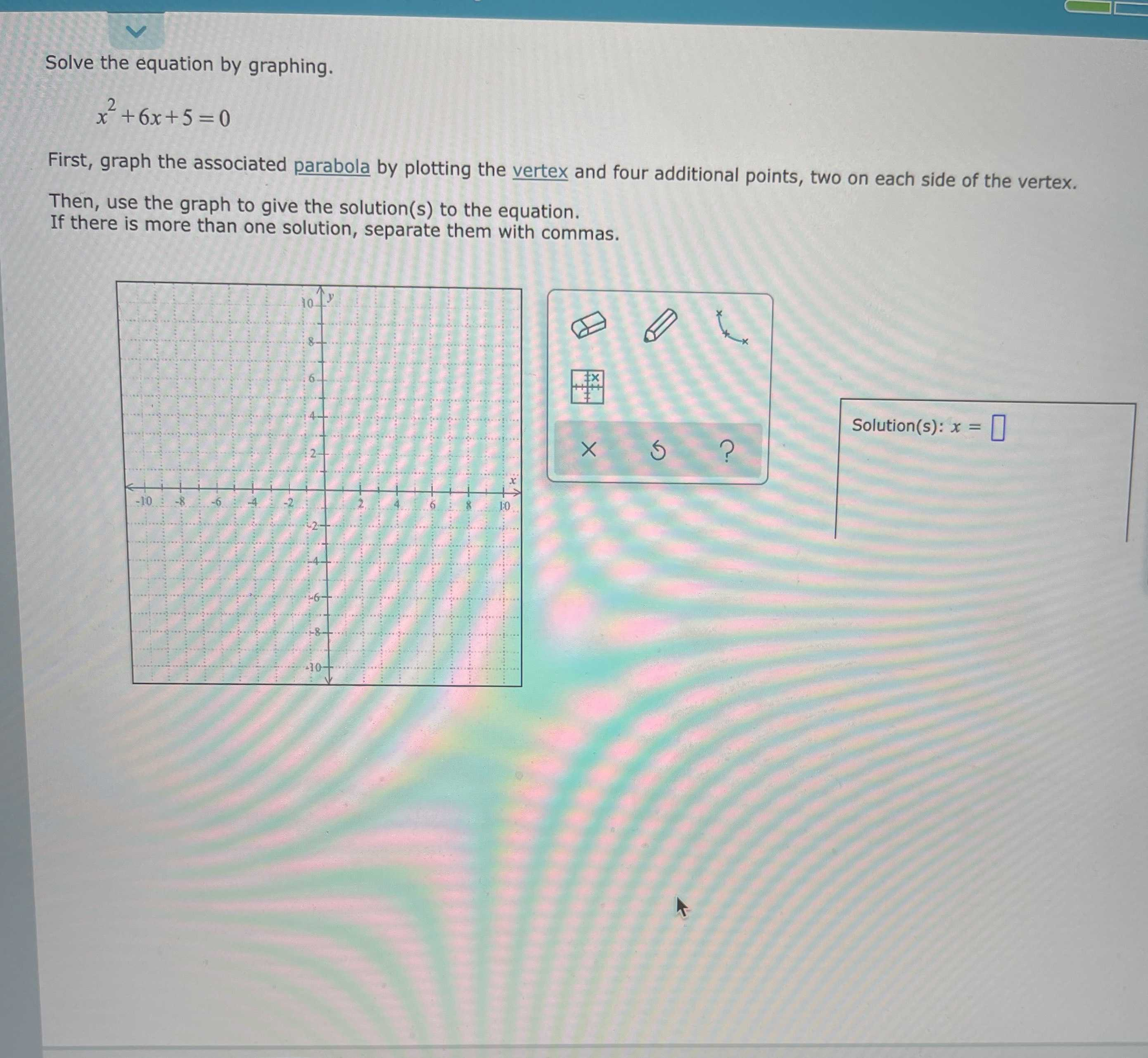### Still have math questions?

Algebra
QuestionSolve the equation by graphing.

$$x ^ { 2 } + 6 x + 5 = 0$$

First, graph the associated parabola by plotting the vertex and four additional points, two on each side of the vertex.

Then, use the graph to give the solution(s) to the equation. If there is more than one solution, separate them with commas.